Tweet   in
 /* styles */ The Dispatch | Vol. 4, No. 2, April 2022
 table div table+table+table div table{width:100%;padding:0}table div table+table+table div table img{width:96.23%;padding:0;float:none}table div table+table+table div table td{width:100%;padding:0 1.88% 18px}/* styles */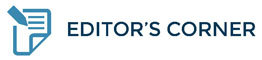# From Everyday Heroes to Heroes for Change

Norman E. Taylor
Editor-in-Chief, Journal of Community Safety and Well-Being

If any of you have recently landed on our Journal homepage, you will have already seen our heartfelt tribute to our colleague Bill Spearn, who lost his battle with cancer last month. Our upcoming Issue 7(2) will also feature an opening guest editorial that will both reflect on Bill’s legacy and hopefully inspire others to continue to carry his torch with renewed commitment to improved CSWB outcomes. I won’t repeat those efforts here. But I will offer personally that it was my distinct honour to have encountered Bill a few years ago, to quickly become his friend and colleague, and to admire the breadth of his commitment and energy to make our world a better place. My thoughts have continued to go out to Bill’s family almost daily since his diagnosis, and I wish them as much comfort in his memory as their grief will allow.

Perhaps it is his recent passing that had me reflecting on the importance of recognizing the incredible sacrifices that all CSWB professionals are required to make, whether in facing the challenges of their day-to-day work, in their always strained work-life balance, or in those times when they emerge as genuine change-makers for the broader system. As we continue to follow our “wellness check” theme, we are encouraged by how often our worldwide contributing Author community revisits these realities in their own submissions, and we look forward to showcasing some of that powerful and instructive content through the coming months. Our call for papers is ongoing.

Many of you may have caught the recent release of the first international textbook dedicated to the still-emerging collaborative policies and practices on which this Journal was founded. The book is titled Law Enforcement and Public Health: Partners for Community Safety and Wellbeing and we extend our congratulations to the editorial team on a truly expansive collection. Together with some of my Journal colleagues and co-authors Cal Corley, Dale McFee and Matt Torigian, it was a proud moment to see our contributed chapter on Improving Community Outcomes through Leveraged Police Leadership included in this seminal work.

Notwithstanding the chapter title, our intent was to trace three distinctive leadership attributes that we believe define the exemplars we have witnessed across the LEPH - CSWB spectrum. And, we make a note that we are addressing ‘leadership’ without the capital ‘L’. In my own view, now more than ever, these characteristics will be in high demand at every level of the human services systems as we all come through some truly difficult times. They will be also vital to attending to the needs of our colleagues as they continue to give of themselves to meet the needs and improve the lives of others.

In our chapter, and as showcased in several case examples drawn from our own experiences over the past several years, we highlight: the courage and passion to expand the mission: the ability to shift focus from positional power to multi-sector influence; and, the vital importance of developing, serving, and supporting others as they pursue their daily duties, and especially whenever they are part of game-changing innovations.

My friend Bill exhibited all of these characteristics and he applied them to achieve important breakthroughs in policy and practice. Every day, I meet more and more professionals committed to learning new ways, to overcoming the inertia that holds back innovation, and to persuasively drawing in others and passing on the courage to expand and update their mission. It falls to all of us to keep them well, as much as we can.

 table div table+table+table+table+table+table div table{width:100%;padding:0}table div table+table+table+table+table+table div table img{width:96.23%;padding:0;float:none}table div table+table+table+table+table+table div table td{width:100%;padding:0 1.88% 18px}/* styles */table.module-6{width:52.64%;padding:0}table div table+table+table+table+table+table+table div table{width:52.64%;float:none;margin-left:auto;margin-right:auto;padding:0}table div table+table+table+table+table+table+table div table a{border:0 none;text-decoration:none}table div table+table+table+table+table+table+table div table img{width:100%!important;border:0 none;text-decoration:none}table div table+table+table+table+table+table+table div table td{width:100%;padding:0}/* styles */
 table div table+table+table+table+table+table+table+table div table{width:100%;padding:0}table div table+table+table+table+table+table+table+table div table img{width:96.23%;padding:0;float:none}table div table+table+table+table+table+table+table+table div table td{width:100%;padding:0 1.88% 18px}/* styles */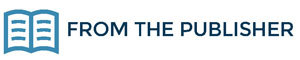# Expanding Reach and Impact Through Indexing

For the Journal of CSWB, the next step to increasing visibility, impact, and readership, is to apply for indexing in reputable scholarly databases. Post-publication distribution through indexing services increases the discoverability of published works, thus increasing the potential to positively impact and improve multi-sector collaborations and overall approaches to CSWB, worldwide.

Outlined below are the key databases and indexing services that SG Publishing will apply to on behalf of the Journal in 2022 and 2023:

▪ Directory of Open Access Journals (DOAJ) – DOAJ is an online directory that indexes and provides access to high quality, open access, peer-reviewed journals. Being indexed in DOAJ means that the journal adheres to high levels of quality in its publishing practices and services to authors and readers.
▪ EBSCOhost & ProQuest – Content aggregator providing comprehensive databases to libraries worldwide, thus exposing the Journal to new readers.
▪ Scopus – Scopus is known for its high level of academic rigor, thus increasing the prestige of the Journal. Inclusion in Scopus will also provide the Journal with a wide range of metrics for indexed content, including a CiteScore.
 ▪ Directory of Open Access Journals (DOAJ) – DOAJ is an online directory that indexes and provides access to high quality, open access, peer-reviewed journals. Being indexed in DOAJ means that the journal adheres to high levels of quality in its publishing practices and services to authors and readers.
 ▪ EBSCOhost & ProQuest – Content aggregator providing comprehensive databases to libraries worldwide, thus exposing the Journal to new readers.
 ▪ Scopus – Scopus is known for its high level of academic rigor, thus increasing the prestige of the Journal. Inclusion in Scopus will also provide the Journal with a wide range of metrics for indexed content, including a CiteScore.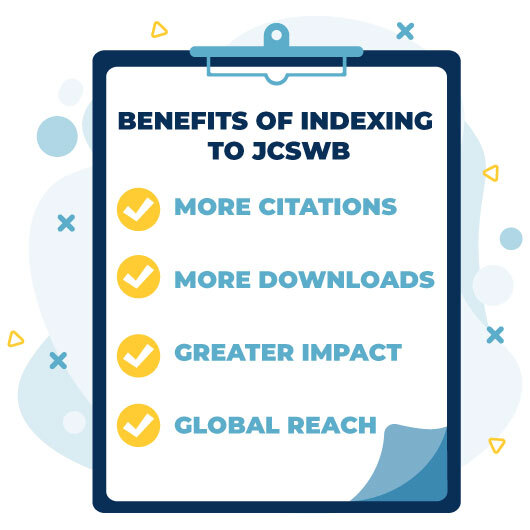/* styles */ We will include updates of the application process and outcomes in future issues of The Dispatch and through the Journal’s social media channels—stay tuned!SG Publishing Inc.
Trusted Scholarly Publisher
www.sgpublishing.ca
@SGPublishingInc

 table div table+table+table+table+table+table+table+table+table+table+table+table+table+table div table{width:100%;padding:0}table div table+table+table+table+table+table+table+table+table+table+table+table+table+table div table img{width:96.23%;padding:0;float:none}table div table+table+table+table+table+table+table+table+table+table+table+table+table+table div table td{width:100%;padding:0 1.88% 18px}/* styles */table.module-14{width:90.57%;padding:0}table div table+table+table+table+table+table+table+table+table+table+table+table+table+table+table div table{width:90.57%;float:none;margin-left:auto;margin-right:auto;padding:0}table div table+table+table+table+table+table+table+table+table+table+table+table+table+table+table div table a{border:0 none;text-decoration:none}table div table+table+table+table+table+table+table+table+table+table+table+table+table+table+table div table img{width:100%!important;border:0 none;text-decoration:none}table div table+table+table+table+table+table+table+table+table+table+table+table+table+table+table div table td{width:100%;padding:0}/* styles */
 table div table+table+table+table+table+table+table+table+table+table+table+table+table+table+table+table div table{width:100%;padding:0}table div table+table+table+table+table+table+table+table+table+table+table+table+table+table+table+table div table img{width:96.23%;padding:0;float:none}table div table+table+table+table+table+table+table+table+table+table+table+table+table+table+table+table div table td{width:100%;padding:0 1.88% 18px}/* styles */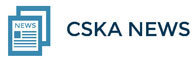# Turning the Age-old Exit Interview to Strategic Advantage

Cal Corley, MBA
CEO, Community Safety Knowledge Alliance

There’s an aspect of knowledge management that has been grating at me for some time – it’s about the vast amounts of important and often specialized knowledge that vanishes with each retirement or departure from key roles across the community safety and well-being system.

The knowledge created in human service organizations represents strategic value. One of the major challenges organizations face today is in translating such knowledge into strategic or competitive advantage.

The concerns I share with so many others are not so much about the explicit knowledge (e.g., reports, data sets, papers etc.) that organizations create. Rather, it’s the tacit knowledge organizations have, but let go of without much thought. Tacit knowledge is what employees know and understand, but that has not been documented or otherwise codified. It is what we know, what we know how to do, what we understand works and what doesn’t work.

In an age marked by knowledge management, no knowledge asset has been as neglected as the tacit knowledge employees create over the course of careers. Imagine the insights and know-how that disappears when a seasoned professional or executive retires or leaves an organization. The deep insights into strategic relationships, the internal challenges and opportunities and potential ways to improve business processes or enhance competitive advantage, are but a few of the knowledge that is typically lost. And how much did the organization invest in developing this tacit knowledge over the course of a career?

Exit interviews have been a traditional approach to gathering such information. Sadly, most exit interview programs fall well short of what is needed to capture the important tacit knowledge that can otherwise benefit the organization going forward. Most organizations focus their exit interviews too narrowly and rely on internal managers or human resource specialists to conduct them – candour suffers.

Organizations interested in getting deep and rich insights have their top performers and executives exit-interviewed either just before or within two weeks of departure. Interviews of those within this group should be conducted by a seasoned interviewer independent of the organization to assure a high degree of candour. It is this group that is likely to have the most insight and perspective, and it is others like them your organization will surely want to attract and/or retain. Four key areas should be explored:

1. HR practices – what is working well and what is not
2. Perceptions of the workplace culture
3. Management effectiveness – strategic and tactical
4. Ideas for improvement
 1 HR practices – what is working well and what is not
 2 Perceptions of the workplace culture
 3 Management effectiveness – strategic and tactical
 4 Ideas for improvement

Something all organizations can’t afford not to be doing – and doing well.

 table div table+table+table+table+table+table+table+table+table+table+table+table+table+table+table+table+table+table+table div table{width:100%;padding:0}table div table+table+table+table+table+table+table+table+table+table+table+table+table+table+table+table+table+table+table div table img{width:96.23%;padding:0;float:none}table div table+table+table+table+table+table+table+table+table+table+table+table+table+table+table+table+table+table+table div table td{width:100%;padding:0 1.88% 18px}/* styles */## In MemoriamThe Journal of CSWB team sadly lost a valued member with the recent passing of Supt. Bill Spearn after a courageous battle with cancer. We owe a great debt of gratitude for Bill’s contribution to the Journal as a Contributing Editor, Reviewer, and Author. His passion, dedication, and expertise will be sorely missed. Our deepest sympathies and thoughts go to his family, friends, and colleagues. Read the full tribute to our colleague here.

 table div table+table+table+table+table+table+table+table+table+table+table+table+table+table+table+table+table+table+table+table+table+table div table{width:100%;padding:0}table div table+table+table+table+table+table+table+table+table+table+table+table+table+table+table+table+table+table+table+table+table+table div table img{width:96.23%;padding:0;float:none}table div table+table+table+table+table+table+table+table+table+table+table+table+table+table+table+table+table+table+table+table+table+table div table td{width:100%;padding:0 1.88% 18px}/* styles */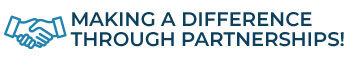/* styles */ The Journal is proud to have partnerships with key organizations within Canada and globally, which are designed to increase awareness for our respective activities. As such, we are pleased to share and highlight relevant information from our partners to our Journal community.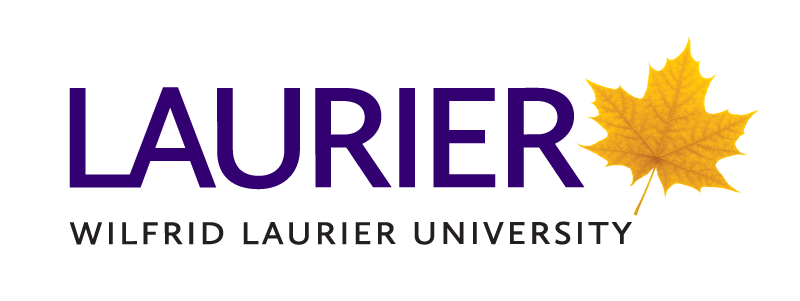# Application Deadline Dates for Fall Start 2022

100% Online Public Safety Degrees | Earn your degree while you work

Program Application Deadlines for September 2022 Start Term:

▪ Honours Bachelor of Arts in Policing - August 17th, 2022
▪ Combined Honours Bachelor of Arts in Criminology and Policing - August 17th, 2022
▪ Master of Public Safety - June 15th, 2022
▪ Countering Crime Graduate Diploma - June 15th, 2022
▪ National Security Graduate Diploma - June 15th, 2022
▪ Emergency Management Graduate Diploma - June 15th, 2022
▪ Border Strategies Graduate Diploma - June 15th, 2022
▪ Public Safety GIS and Data Analytics Graduate Diploma - June 15th, 2022
 ▪ Honours Bachelor of Arts in Policing - August 17th, 2022
 ▪ Combined Honours Bachelor of Arts in Criminology and Policing - August 17th, 2022
 ▪ Master of Public Safety - June 15th, 2022
 ▪ Countering Crime Graduate Diploma - June 15th, 2022
 ▪ National Security Graduate Diploma - June 15th, 2022
 ▪ Emergency Management Graduate Diploma - June 15th, 2022
 ▪ Border Strategies Graduate Diploma - June 15th, 2022
 ▪ Public Safety GIS and Data Analytics Graduate Diploma - June 15th, 2022

Visit www.laurierpublicsafety.ca

To find out how you can set up a Laurier information session for your members, contact:
Maggie Paoletta
Director of Employer Partnerships
Tel. (416) 889-0700

 table div table+table+table+table+table+table+table+table+table+table+table+table+table+table+table+table+table+table+table+table+table+table+table+table+table+table+table div table{width:100%;padding:0}table div table+table+table+table+table+table+table+table+table+table+table+table+table+table+table+table+table+table+table+table+table+table+table+table+table+table+table div table img{width:96.23%;padding:0;float:none}table div table+table+table+table+table+table+table+table+table+table+table+table+table+table+table+table+table+table+table+table+table+table+table+table+table+table+table div table td{width:100%;padding:0 1.88% 18px}/* styles */# OACP’s #ValTags4Ukraine Fundraiser

The Ontario Association of Chiefs of Police (OACP) continues to support victims of the conflict in Ukraine through a fundraising initiative called #ValTags4Ukraine that encourages Ontario vehicle owners to donate their provincial licence plate renewal (validation tags) refunds to help victims of the on-going war. All proceeds raised will go directly to supporting people in Canada displaced due to the war in Ukraine:

▪ Red Cross donation website (English)
▪ Red Cross donation website (French)
 ▪ Red Cross donation website (English)
 ▪ Red Cross donation website (French)
 table div table+table+table+table+table+table+table+table+table+table+table+table+table+table+table+table+table+table+table+table+table+table+table+table+table+table+table+table+table+table div table{width:100%;padding:0}table div table+table+table+table+table+table+table+table+table+table+table+table+table+table+table+table+table+table+table+table+table+table+table+table+table+table+table+table+table+table div table img{width:96.23%;padding:0;float:none}table div table+table+table+table+table+table+table+table+table+table+table+table+table+table+table+table+table+table+table+table+table+table+table+table+table+table+table+table+table+table div table td{width:100%;padding:0 1.88% 18px}/* styles */table div table+table+table+table+table+table+table+table+table+table+table+table+table+table+table+table+table+table+table+table+table+table+table+table+table+table+table+table+table+table+table div table td,table.module-30{width:100%;padding:0}table div table+table+table+table+table+table+table+table+table+table+table+table+table+table+table+table+table+table+table+table+table+table+table+table+table+table+table+table+table+table+table div table{width:100%;float:none;margin-left:auto;margin-right:auto;padding:0}table div table+table+table+table+table+table+table+table+table+table+table+table+table+table+table+table+table+table+table+table+table+table+table+table+table+table+table+table+table+table+table div table a{border:0 none;text-decoration:none}table div table+table+table+table+table+table+table+table+table+table+table+table+table+table+table+table+table+table+table+table+table+table+table+table+table+table+table+table+table+table+table div table img{width:100%!important;border:0 none;text-decoration:none}/* styles */
 table div table+table+table+table+table+table+table+table+table+table+table+table+table+table+table+table+table+table+table+table+table+table+table+table+table+table+table+table+table+table+table+table div table{width:100%;padding:0}table div table+table+table+table+table+table+table+table+table+table+table+table+table+table+table+table+table+table+table+table+table+table+table+table+table+table+table+table+table+table+table+table div table img{width:96.23%;padding:0;float:none}table div table+table+table+table+table+table+table+table+table+table+table+table+table+table+table+table+table+table+table+table+table+table+table+table+table+table+table+table+table+table+table+table div table td{width:100%;padding:0 1.88% 18px}/* styles */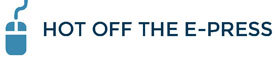https://journalcswb.ca/index.php/cswb/issue/view/23

# Highlights from this issue include:

Time for a wellness check: Looking in on the system, the responder, and the family
[Editorial]
Linna Tam-Seto

Duante Wright and Kim Potter—The chilling effect of death and conviction
[Commentary]
Matt Torigian

Education, preparation, and moral obligation: An examination of hospital employee role in active shooter training response
[Original Research]
McKenzie Wood and Mallory Darais

Offenders on judicial orders: Implications for evidence-based risk management in policing
[Original Research]
Sandy Jung and Gregory Kitura

 table div table+table+table+table+table+table+table+table+table+table+table+table+table+table+table+table+table+table+table+table+table+table+table+table+table+table+table+table+table+table+table+table+table+table+table div table{width:100%;padding:0}table div table+table+table+table+table+table+table+table+table+table+table+table+table+table+table+table+table+table+table+table+table+table+table+table+table+table+table+table+table+table+table+table+table+table+table div table img{width:96.23%;padding:0;float:none}table div table+table+table+table+table+table+table+table+table+table+table+table+table+table+table+table+table+table+table+table+table+table+table+table+table+table+table+table+table+table+table+table+table+table+table div table td{width:100%;padding:0 1.88% 18px}/* styles */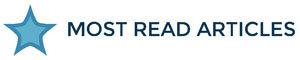/* styles */ Check out our Top 3 most read articles for the Journal of CSWB based on the number of full text views and downloads recorded on our website from April 1, 2021 – Mach 31, 2022. Click here to view other articles from our archives. COVID-19 and the impact on police services (9,109 Views) Katy Kamkar and Shahin Mehdizadeh Vol 5, No. 2 (2020) Policing of sex work in South Africa: The positive policing partnership approach (6,600 Views) Donna Maree Evans, Marlise L. Richter, and Munyaradazi I. Katumba Vol 4, No 4 (2019) Mental health and well-being of police in a health pandemic: Critical issues for police leaders in a post-COVID-19 environment (2,667 Views) Jacqueline M. Drew and Sherri Martin Vol. 5 No. 2 (2020)
 table div table+table+table+table+table+table+table+table+table+table+table+table+table+table+table+table+table+table+table+table+table+table+table+table+table+table+table+table+table+table+table+table+table+table+table+table+table+table div table{width:100%;padding:0}table div table+table+table+table+table+table+table+table+table+table+table+table+table+table+table+table+table+table+table+table+table+table+table+table+table+table+table+table+table+table+table+table+table+table+table+table+table+table div table img{width:96.23%;padding:0;float:none}table div table+table+table+table+table+table+table+table+table+table+table+table+table+table+table+table+table+table+table+table+table+table+table+table+table+table+table+table+table+table+table+table+table+table+table+table+table+table div table td{width:100%;padding:0 1.88% 18px}/* styles */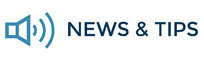# Envisaging Healthy and Safe Communities: Worldwide Lessons in Police and Public Health Partnerships - Special Issue Coming July 2022

A special issue of the Journal of CSWB dedicated to the research derived from the Envisaging the Future project, will be published in July 2022. Published through the support received from Deloitte Inc. and in partnership with the Global Law Enforcement and Public Health Association, this issue will explore progressive community safety strategies worldwide and feature researchers from Africa, Asia, Eastern Europe, Latin America, North America, and Western Europe. Learn more.

# You Can Find Us Here Too!

 table div table+table+table+table+table+table+table+table+table+table+table+table+table+table+table+table+table+table+table+table+table+table+table+table+table+table+table+table+table+table+table+table+table+table+table+table+table+table+table+table+table+table div table,table.module-41{width:48.87%;float:left;padding:0}table div table+table+table+table+table+table+table+table+table+table+table+table+table+table+table+table+table+table+table+table+table+table+table+table+table+table+table+table+table+table+table+table+table+table+table+table+table+table+table+table+table+table div table a{border:0 none;text-decoration:none}table div table+table+table+table+table+table+table+table+table+table+table+table+table+table+table+table+table+table+table+table+table+table+table+table+table+table+table+table+table+table+table+table+table+table+table+table+table+table+table+table+table+table div table img{width:100%!important;border:0 none;text-decoration:none}table div table+table+table+table+table+table+table+table+table+table+table+table+table+table+table+table+table+table+table+table+table+table+table+table+table+table+table+table+table+table+table+table+table+table+table+table+table+table+table+table+table+table div table td{width:100%;padding:0 20px 20px 0}/* styles */ The Journal is proud to be highlighted on the Ontario Association of Chiefs of Police (OACP) website. If you are looking for opportunities to share and pursue the latest research and ideas in the field of community safety, visit us on the OACP’s website to learn more.

# Help us Expand the Reach and Impact of the JCSWB

 table div table+table+table+table+table+table+table+table+table+table+table+table+table+table+table+table+table+table+table+table+table+table+table+table+table+table+table+table+table+table+table+table+table+table+table+table+table+table+table+table+table+table+table+table div table,table.module-43{width:51.13%;float:right;padding:0}table div table+table+table+table+table+table+table+table+table+table+table+table+table+table+table+table+table+table+table+table+table+table+table+table+table+table+table+table+table+table+table+table+table+table+table+table+table+table+table+table+table+table+table+table div table a{border:0 none;text-decoration:none}table div table+table+table+table+table+table+table+table+table+table+table+table+table+table+table+table+table+table+table+table+table+table+table+table+table+table+table+table+table+table+table+table+table+table+table+table+table+table+table+table+table+table+table+table div table img{width:100%!important;border:0 none;text-decoration:none}table div table+table+table+table+table+table+table+table+table+table+table+table+table+table+table+table+table+table+table+table+table+table+table+table+table+table+table+table+table+table+table+table+table+table+table+table+table+table+table+table+table+table+table+table div table td{width:100%;padding:0 0 20px 20px}/* styles */ We invite you to help us support the Journal’s mission by informing your peers, colleagues, and professional network about how they can contribute to and benefit from the growing knowledge base of collaborative solutions for CSWB. To assist with your efforts, we encourage you to distribute our at-a-glance one-page document that highlights key statistics and facts about the Journal: https://journalcswb.ca/index.php/cswb/JCSWBFactSheet

# Quick Search Using the Keyword Tag Cloud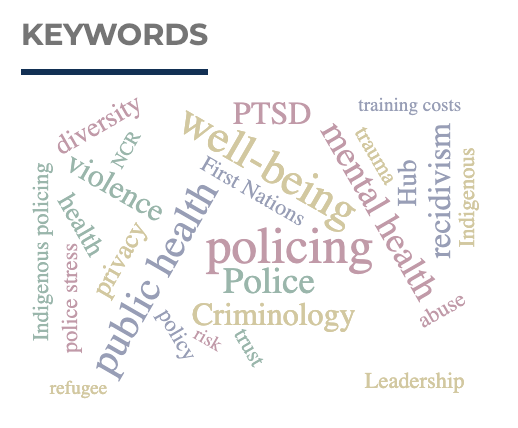The keyword tag cloud can be found on the Journal’s right sidebar and displays the most popular article keywords. Clicking on a keyword displays all articles from the Journal that match that keyword. Looking for a more in-depth search? Search our full article archive here.

 table div table+table+table+table+table+table+table+table+table+table+table+table+table+table+table+table+table+table+table+table+table+table+table+table+table+table+table+table+table+table+table+table+table+table+table+table+table+table+table+table+table+table+table+table+table+table+table div table{width:100%;padding:0}table div table+table+table+table+table+table+table+table+table+table+table+table+table+table+table+table+table+table+table+table+table+table+table+table+table+table+table+table+table+table+table+table+table+table+table+table+table+table+table+table+table+table+table+table+table+table+table div table img{width:96.23%;padding:0;float:none}table div table+table+table+table+table+table+table+table+table+table+table+table+table+table+table+table+table+table+table+table+table+table+table+table+table+table+table+table+table+table+table+table+table+table+table+table+table+table+table+table+table+table+table+table+table+table+table div table td{width:100%;padding:0 1.88% 18px}/* styles */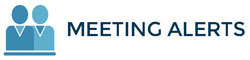table div table+table+table+table+table+table+table+table+table+table+table+table+table+table+table+table+table+table+table+table+table+table+table+table+table+table+table+table+table+table+table+table+table+table+table+table+table+table+table+table+table+table+table+table+table+table+table+table+table+table div table{width:100%;padding:0}table div table+table+table+table+table+table+table+table+table+table+table+table+table+table+table+table+table+table+table+table+table+table+table+table+table+table+table+table+table+table+table+table+table+table+table+table+table+table+table+table+table+table+table+table+table+table+table+table+table+table div table img{width:96.23%;padding:0;float:none}table div table+table+table+table+table+table+table+table+table+table+table+table+table+table+table+table+table+table+table+table+table+table+table+table+table+table+table+table+table+table+table+table+table+table+table+table+table+table+table+table+table+table+table+table+table+table+table+table+table+table div table td{width:100%;padding:0 1.88% 18px}/* styles */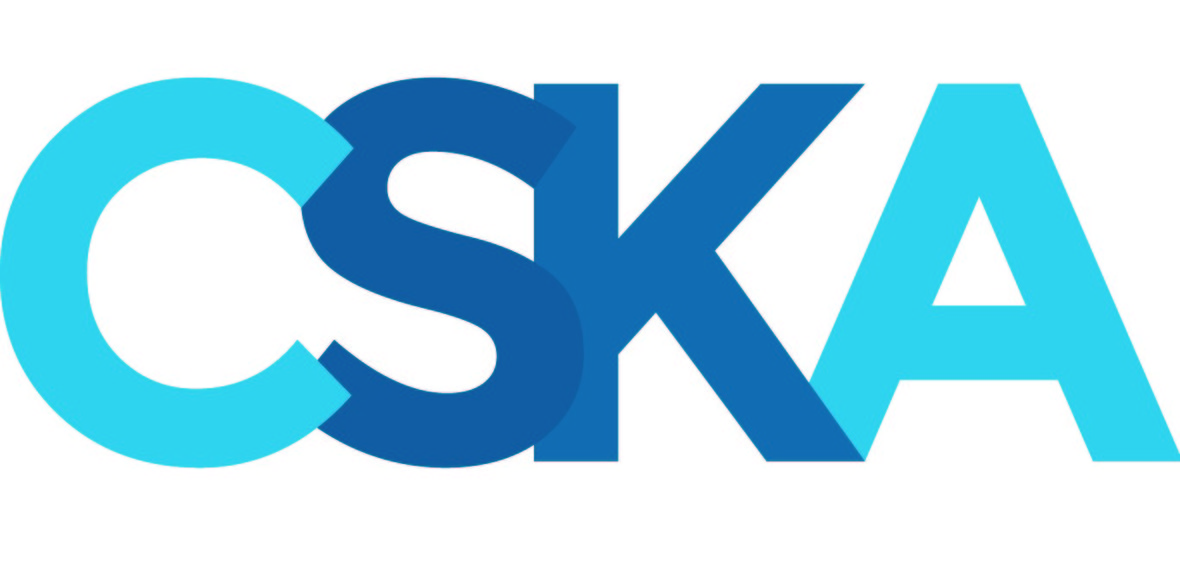table div table+table+table+table+table+table+table+table+table+table+table+table+table+table+table+table+table+table+table+table+table+table+table+table+table+table+table+table+table+table+table+table+table+table+table+table+table+table+table+table+table+table+table+table+table+table+table+table+table+table+table+table+table div table{width:100%;padding:0}table div table+table+table+table+table+table+table+table+table+table+table+table+table+table+table+table+table+table+table+table+table+table+table+table+table+table+table+table+table+table+table+table+table+table+table+table+table+table+table+table+table+table+table+table+table+table+table+table+table+table+table+table+table div table img{width:96.23%;padding:0;float:none}table div table+table+table+table+table+table+table+table+table+table+table+table+table+table+table+table+table+table+table+table+table+table+table+table+table+table+table+table+table+table+table+table+table+table+table+table+table+table+table+table+table+table+table+table+table+table+table+table+table+table+table+table+table div table td{width:100%;padding:0 1.88% 18px}/* styles */